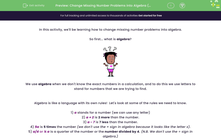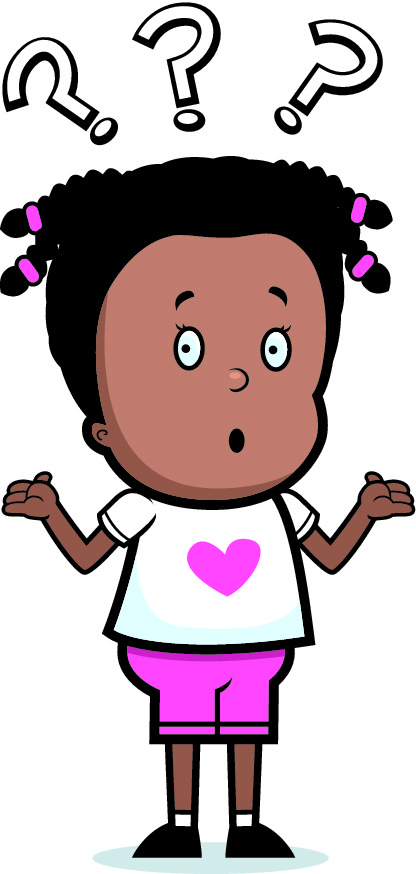# Use Algebra to Express Mathematical Problems

In this worksheet, students will express missing number problems algebraically.Key stage:  KS 2

Curriculum topic:   Algebra

Curriculum subtopic:   Express Problems Algebraically

Difficulty level:#### Worksheet Overview

In this activity, we'll be learning how to change missing number problems into algebra.

So first... what is algebra?We use algebra when we don't know the exact numbers in a calculation, and to do this we use letters to stand for numbers that we are trying to find.

Algebra is like a language with its own rules!  Let's look at some of the rules we need to know.

1) a stands for a number (we can use any letter)

2) a + 2 is 2 more than the number.

3) a - 7 is 7 less than the number.

4) 5a is 5 times the number (we don't use the × sign in algebra because it looks like the letter x).

5) a/4 or ¼ a is a quarter of the number or the number divided by 4. (N.B. We don't use the ÷ sign in algebra.)

Let's now use our knowledge to work out the following problem:

I think of a number.

1)  I double it.

2) Take away 5.

3) I get 21.

Write this using algebra.

Use n for the number I think of.

Solution

n is the number I think of.

1) Double it to get 2n (remember this means 2 times n)

2) Take away 5 to get 2n - 5

3) This equals 21, so we write:

2n - 5 = 21

Do you get it now?Let's have a go at some questions then!

### What is EdPlace?

We're your National Curriculum aligned online education content provider helping each child succeed in English, maths and science from year 1 to GCSE. With an EdPlace account you’ll be able to track and measure progress, helping each child achieve their best. We build confidence and attainment by personalising each child’s learning at a level that suits them.

Get started